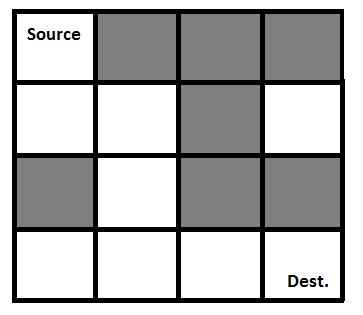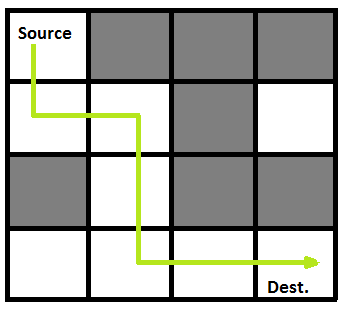# C Program for Rat in a Maze | Backtracking-2

We have discussed Backtracking and Knight’s tour problem in Set 1. Let us discuss Rat in a Maze as another example problem that can be solved using Backtracking.

A Maze is given as N*N binary matrix of blocks where source block is the upper left most block i.e., maze and destination block is lower rightmost block i.e., maze[N-1][N-1]. A rat starts from source and has to reach the destination. The rat can move only in two directions: forward and down.
In the maze matrix, 0 means the block is a dead end and 1 means the block can be used in the path from source to destination. Note that this is a simple version of the typical Maze problem. For example, a more complex version can be that the rat can move in 4 directions and a more complex version can be with a limited number of moves.

Following is an example maze.

` Gray blocks are dead ends (value = 0). `Following is binary matrix representation of the above maze.

```                {1, 0, 0, 0}
{1, 1, 0, 1}
{0, 1, 0, 0}
{1, 1, 1, 1}
```

Following is a maze with highlighted solution path.Following is the solution matrix (output of program) for the above input matrx.

```                {1, 0, 0, 0}
{1, 1, 0, 0}
{0, 1, 0, 0}
{0, 1, 1, 1}
All enteries in solution path are marked as 1.
```

## C/C++

 `/* C/C++ program to solve Rat in a Maze problem using ` `   ``backtracking */` `#include ` ` `  `// Maze size ` `#define N 4 ` ` `  `bool` `solveMazeUtil(``int` `maze[N][N], ``int` `x, ``int` `y, ``int` `sol[N][N]); ` ` `  `/* A utility function to print solution matrix sol[N][N] */` `void` `printSolution(``int` `sol[N][N]) ` `{ ` `    ``for` `(``int` `i = 0; i < N; i++) { ` `        ``for` `(``int` `j = 0; j < N; j++) ` `            ``printf``(``" %d "``, sol[i][j]); ` `        ``printf``(``"\n"``); ` `    ``} ` `} ` ` `  `/* A utility function to check if x, y is valid index for N*N maze */` `bool` `isSafe(``int` `maze[N][N], ``int` `x, ``int` `y) ` `{ ` `    ``// if (x, y outside maze) return false ` `    ``if` `(x >= 0 && x < N && y >= 0 && y < N && maze[x][y] == 1) ` `        ``return` `true``; ` ` `  `    ``return` `false``; ` `} ` ` `  `/* This function solves the Maze problem using Backtracking.  It mainly ` `   ``uses solveMazeUtil() to solve the problem. It returns false if no  ` `   ``path is possible, otherwise return true and prints the path in the ` `   ``form of 1s. Please note that there may be more than one solutions,  ` `   ``this function prints one of the feasible solutions.*/` `bool` `solveMaze(``int` `maze[N][N]) ` `{ ` `    ``int` `sol[N][N] = { { 0, 0, 0, 0 }, ` `                      ``{ 0, 0, 0, 0 }, ` `                      ``{ 0, 0, 0, 0 }, ` `                      ``{ 0, 0, 0, 0 } }; ` ` `  `    ``if` `(solveMazeUtil(maze, 0, 0, sol) == ``false``) { ` `        ``printf``(``"Solution doesn't exist"``); ` `        ``return` `false``; ` `    ``} ` ` `  `    ``printSolution(sol); ` `    ``return` `true``; ` `} ` ` `  `/* A recursive utility function to solve Maze problem */` `bool` `solveMazeUtil(``int` `maze[N][N], ``int` `x, ``int` `y, ``int` `sol[N][N]) ` `{ ` `    ``// if (x, y is goal) return true ` `    ``if` `(x == N - 1 && y == N - 1) { ` `        ``sol[x][y] = 1; ` `        ``return` `true``; ` `    ``} ` ` `  `    ``// Check if maze[x][y] is valid ` `    ``if` `(isSafe(maze, x, y) == ``true``) { ` `        ``// mark x, y as part of solution path ` `        ``sol[x][y] = 1; ` ` `  `        ``/* Move forward in x direction */` `        ``if` `(solveMazeUtil(maze, x + 1, y, sol) == ``true``) ` `            ``return` `true``; ` ` `  `        ``/* If moving in x direction doesn't give solution then ` `           ``Move down in y direction  */` `        ``if` `(solveMazeUtil(maze, x, y + 1, sol) == ``true``) ` `            ``return` `true``; ` ` `  `        ``/* If none of the above movements work then BACKTRACK:  ` `            ``unmark x, y as part of solution path */` `        ``sol[x][y] = 0; ` `        ``return` `false``; ` `    ``} ` ` `  `    ``return` `false``; ` `} ` ` `  `// driver program to test above function ` `int` `main() ` `{ ` `    ``int` `maze[N][N] = { { 1, 0, 0, 0 }, ` `                       ``{ 1, 1, 0, 1 }, ` `                       ``{ 0, 1, 0, 0 }, ` `                       ``{ 1, 1, 1, 1 } }; ` ` `  `    ``solveMaze(maze); ` `    ``return` `0; ` `} `

Output:

```1  0  0  0
1  1  0  0
0  1  0  0
0  1  1  1
```

Please refer complete article on Rat in a Maze | Backtracking-2 for more details!

My Personal Notes arrow_drop_up

Article Tags :

Be the First to upvote.

Please write to us at contribute@geeksforgeeks.org to report any issue with the above content.